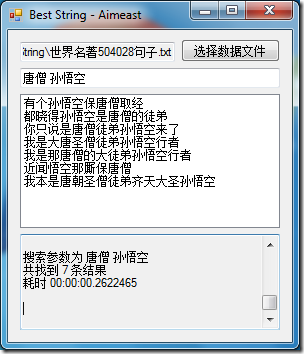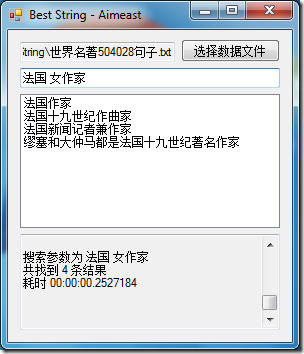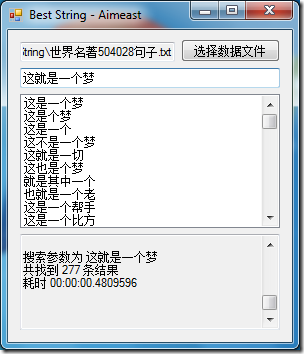# 【算法】字符串近似搜索

来源：.Net.NewLife。
需求：假设在某系统存储了许多地址，例如：“北京市海淀区中关村大街1号海龙大厦”。用户输入“北京 海龙大厦”即可查询到这条结果。另外还需要有容错设计，例如输入“广西 京岛风景区”能够搜索到"广西壮族自治区京岛风景名胜"。最终的需求是：可以根据用户输入，匹配若干条近似结果共用户选择
目的：避免用户输入类似地址导致数据出现重复项。例如，已经存在“北京市中关村”，就不应该再允许存在“北京中关村”。

举例此类技术在搜索引擎中早已广泛使用，例如“查询预测”功能。

要实现此算法，首先需要明确“字符串近似”的概念。

计算字符串相似度通常使用的是动态规划(DP)算法。

常用的算法是 Levenshtein Distance。用这个算法可以直接计算出两个字符串的“编辑距离”。所谓编辑距离，是指一个字符串，每次只能通过插入一个字符、删除一个字符或者修改一个字符的方法，变成另外一个字符串的最少操作次数。这就引出了第一种方法：计算两个字符串之间的编辑距离。稍加思考之后发现，不能用输入的关键字直接与句子做匹配。你必须从句子中选取合适的长度后再做匹配。把结果按照距离升序排序。

using System;
using System.Collections.Generic;
using System.Linq;
using System.Text;

namespace BestString
{
public static class SearchHelper
{
public static string[] Search(string param, string[] datas)
{
if (string.IsNullOrWhiteSpace(param))
return new string;

string[] words = param.Split(new char[] { ' ', '　' }, StringSplitOptions.RemoveEmptyEntries);

foreach (string word in words)
{
int maxDist = (word.Length - 1) / 2;

var q = from str in datas
where word.Length <= str.Length
&& Enumerable.Range(0, maxDist + 1)
.Any(dist =>
{
return Enumerable.Range(0, Math.Max(str.Length - word.Length - dist + 1, 0))
.Any(f =>
{
return Distance(word, str.Substring(f, word.Length + dist)) <= maxDist;
});
})
orderby str
select str;
datas = q.ToArray();
}

return datas;
}

static int Distance(string str1, string str2)
{
int n = str1.Length;
int m = str2.Length;
int[,] C = new int[n + 1, m + 1];
int i, j, x, y, z;
for (i = 0; i <= n; i++)
C[i, 0] = i;
for (i = 1; i <= m; i++)
C[0, i] = i;
for (i = 0; i < n; i++)
for (j = 0; j < m; j++)
{
x = C[i, j + 1] + 1;
y = C[i + 1, j] + 1;
if (str1[i] == str2[j])
z = C[i, j];
else
z = C[i, j] + 1;
C[i + 1, j + 1] = Math.Min(Math.Min(x, y), z);
}
return C[n, m];
}
}
}


分析这个方法后发现，每次对一个句子进行相关度比较的时候，都要把把句子从头到尾扫描一次，每次扫描还需要以最大误差作长度控制。这样一来，对每个句子的计算次数大大增加。达到了二次方的规模（忽略距离计算时间）。

所以我们需要更高效的计算策略。在纸上写出一个句子，再写出几个关键字。一个一个涂画之后，偶然发现另一种字符串相关的算法完全可以适用。那就是 Longest common subsequence（LCS，最长公共字串）。为什么这个算法可以用来计算两个字符串的相关度？先看一个例子：

这里用了两个关键字进行搜索。可以看出来两个关键字都有部分匹配了句子中的若干部分。这样可以单独为两个关键字计算 LCS，LCS之和就是简单的相关度。看到这里，你若是已经理解了核心思想，已经可以实现出基本框架了。但是，请看下面这个例子：

看出来问题了吗？下面还是使用同样的关键字和句子。

举这个例子为了说明，在进行 LCS 计算的过程中，得到的结果并不能保证就是我们期望的结果。为了①保证所匹配的结果中不存在交集，并且②在句子中的匹配结果尽可能的短，需要采取两个补救措施。（为什么需要满足这样的条件，读者自行思考）

第一：可以在单次计算 LCS 之后，用贪心策略向前（向后）找到最先能够完成匹配的位置，再用相同的策略向后（向前）扫描。这样可以满足第二个条件找到句子中最短的匹配。如果你对 LCS 算法有深入了解，完全可以在计算 LCS 的过程中找到最短匹配的结束位置，然后只需要进行一次向前扫描就可以完成。这样节约了一次扫描过程。

第二：增加一个标记数组，记录句子中的字符是否被匹配过。

最后标记数组中标记过的位置就是匹配结果。

相信你看到这里一定非常头晕，下面用一个例子解释：（句子）

A   B C   D

A   B C D

你可能会匹配成 AYABZCBXCDD，AYABZCBXCD，ABZCBXCDD，ABZCBXCD。我们实际需要的只是ABZCBXCD。

使用LCS匹配之后，得到的很可能是 XAYABZCBXCDDYZ；

用贪心策略向前处理后，得到结果为 XAYABZCBXCDDYZ；

用贪心策略向后处理后，得到结果为 XAYABZCBXCDDYZ。

这样处理的目的是为了避免得到较长的匹配结果（类似正则表达式的贪婪、懒惰模式）。

以上只是描述了怎么计算两个字符串的相似程度。除此之外还需要：①剔除相似度较低的结果；②对结果进行排序。

剔除相似度较低的结果，这里设定了一个阈值：差错比例不能超过匹配结果长度的一半。

对结果进行排序，不能够直接使用相似度进行排序。因为相似度并没有考虑到句子的长度。按照使用习惯，通常会把匹配度高，并且句子长度短的放在前面。这就得到了排序因子：（不匹配度+0.5）/句子长度。

最后得到我们最终的搜索方法

using System;
using System.Collections.Generic;
using System.Linq;
using System.Text;
using System.Diagnostics;

namespace BestString
{
public static class SearchHelper
{
public static string[] Search(string param, string[] items)
{
if (string.IsNullOrWhiteSpace(param) || items == null || items.Length == 0)
return new string;

string[] words = param
.Split(new char[] { ' ', '\u3000' }, StringSplitOptions.RemoveEmptyEntries)
.OrderBy(s => s.Length)
.ToArray();

var q = from sentence in items.AsParallel()
let MLL = Mul_LnCS_Length(sentence, words)
where MLL >= 0
orderby (MLL + 0.5) / sentence.Length, sentence
select sentence;

return q.ToArray();
}

//static int[,] C = new int[100, 100];

/// <summary>
///
/// </summary>
/// <param name="sentence"></param>
/// <param name="words">多个关键字。长度必须大于0，必须按照字符串长度升序排列。</param>
/// <returns></returns>
static int Mul_LnCS_Length(string sentence, string[] words)
{
int sLength = sentence.Length;
int result = sLength;
bool[] flags = new bool[sLength];
int[,] C = new int[sLength + 1, words[words.Length - 1].Length + 1];
//int[,] C = new int[sLength + 1, words.Select(s => s.Length).Max() + 1];
foreach (string word in words)
{
int wLength = word.Length;
int first = 0, last = 0;
int i = 0, j = 0, LCS_L;
//foreach 速度会有所提升，还可以加剪枝
for (i = 0; i < sLength; i++)
for (j = 0; j < wLength; j++)
if (sentence[i] == word[j])
{
C[i + 1, j + 1] = C[i, j] + 1;
if (first < C[i, j])
{
last = i;
first = C[i, j];
}
}
else
C[i + 1, j + 1] = Math.Max(C[i, j + 1], C[i + 1, j]);

LCS_L = C[i, j];
if (LCS_L <= wLength >> 1)
return -1;

while (i > 0 && j > 0)
{
if (C[i - 1, j - 1] + 1 == C[i, j])
{
i--;
j--;
if (!flags[i])
{
flags[i] = true;
result--;
}
first = i;
}
else if (C[i - 1, j] == C[i, j])
i--;
else// if (C[i, j - 1] == C[i, j])
j--;
}

if (LCS_L <= (last - first + 1) >> 1)
return -1;
}

return result;
}
}
}


对于此类问题，要想得到更快速的实现，必须要用到分词+索引的方案。在此不做探讨。

PS：

①由于若干原因，此文写作时间长达半个月之久。写作思维极不连续，不保证任何人都能看懂；

②.Net.NewLife 群中此资源所作限制之日期，此时结束；

③测试所用数据请自行生成。

posted @ 2011-09-05 19:24  Aimeast  阅读(...)  评论(...编辑  收藏